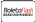Unifying Gravity with the Atomic Scale, Vol. II的封面

# Unifying Gravity with the Atomic Scale, Vol. II

## Rewriting the Foundation of Physics

Scholars' Press (2019-04-14 )有获得代金券的资格
ISBN-13:

### 978-613-8-82847-1

ISBN-10:
613882847X
EAN:
9786138828471

Since the discovery of the massless formula for gravitational acceleration g=τc^2 in 2007, this is the third book in the series rewriting the foundations of physics, This book covers the origins of gravitational fields, gravity modification engine design, black holes and their black particles. It provides an alternative explanation to the Universe expansion red shift data. Hand in hand with this is the rewrite of photon probabilities, how Nature implements probabilities, a better handle on randomness versus probabilities, probability control, probability as a field theory and how gravitational fields deform probability fields.

Scholars' Press

http://www.scholars-press.com

Benjamin T Solomon

248

2019-04-14

557.38 R\$

gravitational constant, Gravity Modification, General Relativity, Special Theory of Relativity, MASS, Spacetime, Black Holes### 链接